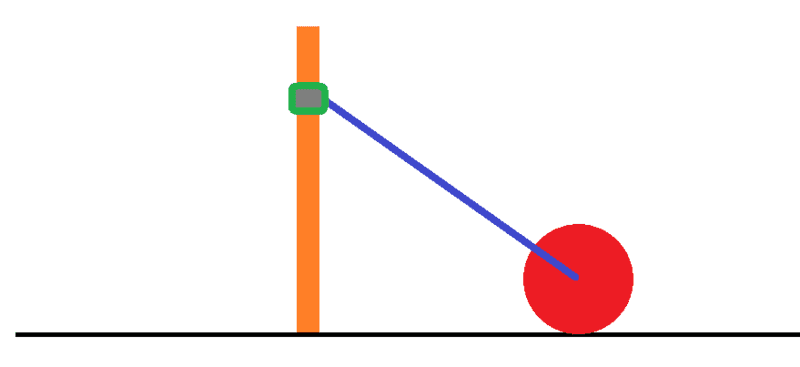# Equation of motion for a simple mechanical system

• I
• davidwinth
In summary, the system has the following properties:The rod has a length of ##L## and a mass of ##m_b##.The disk has a radius of ##R## and a mass of ##m_d##.The collar is free to slide without friction on a vertical and rigid pole.The disk rotates without slipping on the floor.The translational and rotational velocities of the center of mass of the collar are strictly vertical.The total kinetic energy for the system is the sum of all these contributions:$$\frac{1}{2}m_{c}(Lcos(\theta)\dot{\theta})^2 + #### davidwinth TL;DR Summary I want to find an equation of motion for the following system through the use of the Lagrangian approach. The system is shown below. It consists of a rod of length ##L## and mass ##m_b## connecting a disk of radius ##R## and mass ##m_d## to a collar of mass ##m_c## which is in turn free to slide without friction on a vertical and rigid pole. The disk rolls without slipping on the floor. The ends of the rod are attached such that they rotate without friction. I choose the angle ##\theta## between the horizontal and the rod as the generalized coordinate. The velocity of the center of mass of the collar is strictly vertical, and is given by: ##V{_c} = Lcos(\theta)\dot{\theta}##. The velocity of the center of mass of the disk is strictly horizontal, and is given by ##V{_d}=-Lsin(\theta)\dot{\theta}##. The translational velocity of the center of mass of the rod is obtained from its components as: ##V_{b} = \sqrt{\dot{x}_{b}^2 + \dot{y}_{b}^2} = \frac{L\dot{\theta}}{2}##. The rotational velocity of the disk about its center of mass is given as: ##\omega_d = \frac{-Lsin(\theta)\dot{\theta}}{R}##. The rotational velocity of the rod about its center of mass is given as: ##\omega_b = \dot{\theta}##. Thus, the total kinetic energy for this system is the sum of all these contributions:$$\frac{1}{2}m_{c}(Lcos(\theta)\dot{\theta})^2 + \frac{1}{2}m_{b}\left(\frac{L\dot{\theta}}{2}\right)^2 + \frac{1}{2}m_{d}\left(Lsin(\theta)\dot{\theta}\right)^2 + \frac{1}{2}I_{d}\left(\frac{Lsin(\theta)\dot{\theta}}{R}\right)^2 + \frac{1}{2}I_{b}(\dot{\theta})^2

Where the ##I_d## and ##I_b## are the moment of inertia about the centroid of the disk and rod, respectively.

My doubt is whether I have correctly accounted for the motion of the rod by decomposing it this way. Is there something I have neglected in considering the motion of the rod?•vanhees71
davidwinth said:
My doubt is whether I have correctly accounted for the motion of the rod by decomposing it this way. Is there something I have neglected in considering the motion of the rod?
So far, everything looks good to me.

•davidwinth
Thank you!

## 1. What is the equation of motion for a simple mechanical system?

The equation of motion for a simple mechanical system is given by Newton's second law of motion, which states that the net force on an object is equal to its mass multiplied by its acceleration. This can be written as F = ma, where F is the net force acting on the object, m is the mass of the object, and a is the acceleration of the object.

## 2. How is the equation of motion derived?

The equation of motion is derived from Newton's second law of motion, which is based on the fundamental principles of classical mechanics. It can also be derived from the laws of conservation of momentum and energy.

## 3. What is a simple mechanical system?

A simple mechanical system is a physical system that can be described by a set of equations and laws of motion. It typically consists of a single object or a small number of objects that interact with each other through forces such as gravity, friction, or tension.

## 4. What are some examples of simple mechanical systems?

Examples of simple mechanical systems include a swinging pendulum, a bouncing ball, a moving car, or a seesaw. These systems can be described by the equation of motion and other principles of mechanics.

## 5. How is the equation of motion used in real-life applications?

The equation of motion is used in various fields such as engineering, physics, and biomechanics to analyze and predict the behavior of objects in motion. It is also used in designing machines, structures, and vehicles, and in understanding natural phenomena such as the motion of planets and stars.